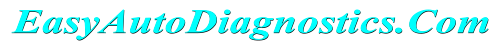If you performed an engine compression test and one or more values were lower than the rest, then there's a good chance that those cylinders are causing a rough idle or a misfire.

To help you find out, I've created a low engine compression calculator to calculate the lowest compression value a cylinder can have before it starts to misfire or cause a rough idle condition.

Contents of this tutorial at a glance:

## Low Compression Calculator

Low Compression Calculator:

Lowest compression value (optional):

Highest compression value (required):

If a compression value varies by more than 15% of the highest compression value, then that engine cylinder will cause a misfire and/or rough idle. What the low compression calculator does is find out what the 15% compression value is compared to the highest compression value you got.

## How To Manually Calculate The Lowest Possible Compression Value

If you'd like to know the math that determines the lowest possible compression value an engine cylinder can have, this is the section for you.

Here's the method I've used to figure it out:

• STEP 1: Multiply the highest compression value by 0.15 (this is the decimal value of 15%).
• STEP 2: Round the result to the nearest one (for example: 25.6 would become 26).
• STEP 3: Subtract the result (the number that was rounded) from the highest compression value.
• ANSWER: The result of this subtraction is the lowest possible compression value the other cylinders can have.

For example, let's say I got the following compression test results (from a four cylinder engine):

Cylinder Pressure
#1 120
#2 165
#3 170
#4 175

Here's the math I would do to calculate the minimum allowable value:

• STEP 1: 175 x 0.15 = 26.25.
• STEP 2: 26.25 = 26 (rounded to nearest one).
• STEP 3: 175 - 26 = 149.
• ANSWER: 149 PSI. Any cylinder with this compression (or lower) value will misfire.

Looking at the table of the compression test results, I can conclude that cylinder #1 is the one causing a misfire due to low engine compression.

## What Should The Normal Compression Value Be For My Vehicle?

It used to be that repair manuals had a factory specification for what the 'normal' cylinder compression should be. Not anymore.

If your online search doesn't turn up a 'good' compression specification for your vehicle, you can calculate an estimate of a 'good' compression value by multiplying your intake pressure (intake manifold vacuum) by your engine's compression ratio.

This involves doing a little research online or in a repair manual to find out the compression ratio of your particular vehicle's engine.

Once you've found the compression ratio, then you multiply it by the intake manifold pressure your vehicle's engine is producing at idle.

For example if a 2009 4.6L Ford F150 has a compression ratio of 9.8:1 and its intake pressure (intake manifold vacuum) is at 17 in.Hg, then you can expect a cylinder pressure in the 170 PSI range (17 x 9.8 = 166.6, which I then round to the nearest 10 and call it 170).

What I do is something a bit different. I know that for most engines, vacuum at idle is usually about 18 to 22 in.Hg (although some may produce only 15 to 17 in.Hg at idle).

I find the engine compression ratio specification and multiply it by 18 (I don't measure intake manifold vacuum). So, let's say I'm working on a 1998 2.4L Nissan Frontier with a compression ratio of 9.2:1. I then do the following math:

• STEP 1: 18 x 9.2 = 165.6
• STEP 2: 165.6 = 170 (rounded to nearest 10) .
• ANSWER: 170 PSI is now my estimated 'good' compression value I should see on each cylinder.If this info really saved the day, buy me a beer!